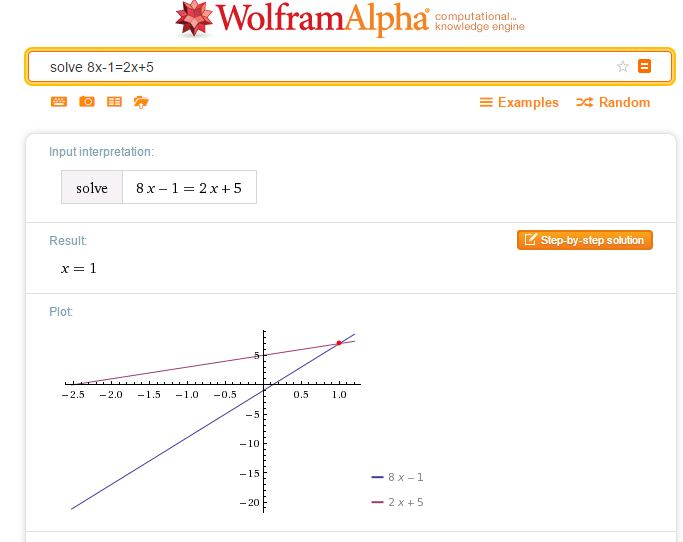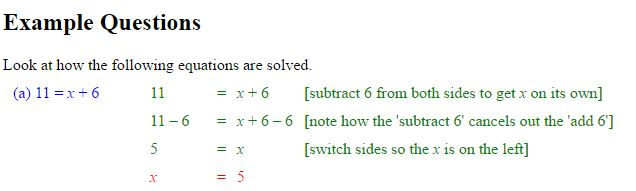# Solving Linear Equations

A collection of resources to use to demonstrate and practise solving equations.

Student Exercises
I find Owen Elton’s worksheet, Balancing Equations on TES Resources very useful when introducing equations, the diagrams emphasise that we must do the same to both sides.
(See Diagrams in Mathematics, for more on the use of diagrams to help understanding).

As an alternative to the balance approach, consider this doing / undoing approach, described here by Don Steward, this is an approach I use for finding inverse functions. The exercises Don refers to are here.

One of the Standards Unit resources, A2 Creating and solving equations (in Mostly Algebra) uses this approach, students create an equation and  then undo it; this is a great exercise for demonstrating notation. Another resource with this approach comes from the Mathematics Assessment Project (the design and development was led by the MARS Shell Center team at the University of Nottingham) Building and Solving Linear Equations lesson

For a superb collection of ideas and student exercises for solving linear equations see all Don Steward’s posts tagged linear equations. Many of these outstanding resources use a very visual approach with very clear diagrams to help students’ understanding.

There are numerous questions on linear equations on Diagnostic Questions.A simple way to check a solution to an equation of any type is to simply enter your equation as a WolframAlpha query. Note that WolframAlpha includes a graphical illustration; it is so important for students to understand how equations may be solved graphically; I always illustrate graphical solutions when we are working with Algebra to help students make these links. Desmos of course, is ideal here.Centre for Innovation in Mathematics TeachingCIMT
have tutorials on equations: Linear Equations 1, Linear Equations 2, and Linear Equations with Brackets.

The following three resources work well for demonstrating the balance method of solving linear equations.

Duncan Keith’s Linear Equation Calculator is available on STEM Learning.Choose the type of equation you require then the sequence of operations required to solve the equation.
Select Do it after each operation, for example -32 Do it were the keys selected to start the above problem.

The slideshow below shows how to use the calculator to solve equations where the unknown is on both sides.

Mathisfun

Mathisfun has this very clear and easy to use interactive illustrating the solution of linear equations.

## One comment on “Solving Linear Equations”

1.mhorley

Lots of good stuff here! I used this recently:
http://flashmaths.co.uk/viewFlash.php?id=26
it’s similar to the Maths is Fun scales but somehow a bit more intuitive (I thought!)

I like the analogy of balances to introduce equations, although I think you need to move away from them when you bring in negatives (balloons don’t do it for me!). Also, I’d be interested to know what order you do things in. My preference is:

1) 3x = 6, x + 5 = 8
2) 3x + 1 = 7, closely followed by
3) 3x = 4 + 2x (using a balance method)
4) Then go on to negatives, e.g. 3x – 1 = 5
5) Then negative solutions
6) Then fractions (after lots of practice)

How do others do it? Is bringing in unknowns on both sides early a good idea or not?